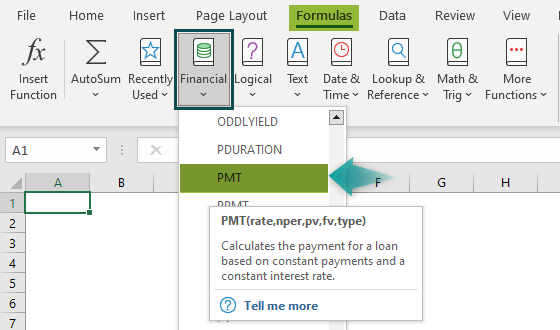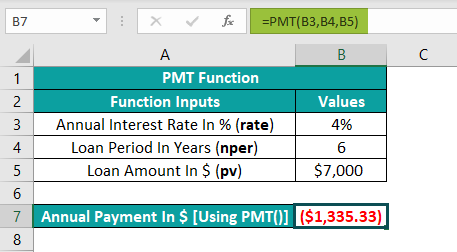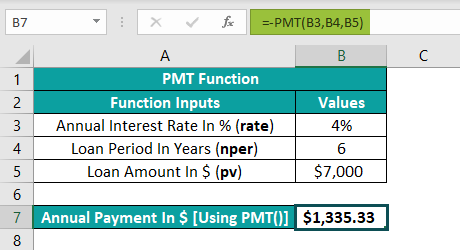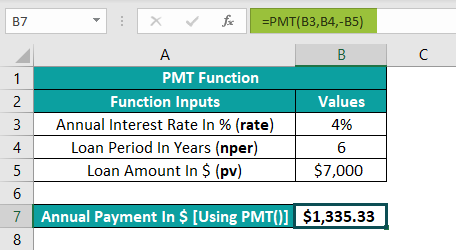# PMT Function in ExcelArticle byExcelMojo Team## What Is PMT Function In Excel?

The PMT function in Excel calculates the total amount of a loan i.e., the principal and the interest, to be paid over a period based on fixed payments and a fixed interest rate. This function calculates weekly and monthly periodic payments.

The PMT excel function is an inbuilt function, so we can enter the formula directly in the cell or choose it from the “Function Library”.

For example, the below table contains the annual interest rate, loan period, and amount details required to determine the payment towards the loan of \$80,000, the interest rate is 6% per year, with annual payment as the loan payment frequency.

Select cell B7, enter the formula =PMT(B3,B4,B5), and press the “Enter” key.

The output is shown above. The PMT excel function in cell B7 returns a negative value, indicating that it is an outgoing payment.

###### Key Takeaways
• The PMT Excel function calculates the total amount we need to pay towards our availed loan over a fixed period and interest rate. And it displays the output in the Currency format.
• The PMT() takes five arguments: rate, nper, pv, fv, and type. While the first three arguments are mandatory, the remaining ones are optional. And if we omit the last two arguments from the PMT(), the function takes their default value of 0 for the loan payment calculations.
• The PMT() formula will vary depending on the loan payment frequency. Therefore, we will have to divide and multiply the arguments, rate, and nper with the number of payments per year while supplying them as input to the PMT().
• The PMT() is useful for developing a loan payment calculator to help users assess the best suitable loan payment options.

### PMT() Excel Formula

The PMT excel formula is:

The PMT function arguments are:

• rate: The loan interest rate. It is a mandatory argument.
• nper: The total number of payments towards the loan. It is a mandatory argument.
• pv: The principal amount or the current value of the sequence of future payments. It is a mandatory argument.
• fv: The future value. It is the balance amount we want to achieve once we make the last payment. If we ignore this value in the PMT excel formula, it takes the default value of 0. It is an optional argument.
• type: It indicates when the payments are due. If we omit the type argument, the PMT() will consider it as 0, interpreting the due payment time as at the end of the payment period. However, if the argument value is 1, the due payment time is at the start of the period. It is an optional argument.

A few critical points to consider while using the PMT function arguments:

• If the rate is less than or equal to -1 or nper is 0, the PMT function returns the #NUM! error.
• If we provide non-numeric arguments as input, we will get the #VALUE! error.
• The PMT function arguments vary according to the loan payment frequency, typically weekly, monthly, quarterly, or annually.
• To determine the weekly, monthly, or quarterly payments, we must convert the annual interest rates and the number of periods in the PMT excel function to weekly, monthly, or quarterly.
• While the data formats of interest rate and loan amount are Percentage and Currency, the PMT() return value’s default format will be Currency.

### How To Use PMT Excel Function?

We can use the PMT Excel Function in 2 methods, namely,

1. Access through the Excel ribbon.
2. Use in the worksheet manually.

[Special Note: First, makes sure the following details are intact.

1. Ensure the input values i.e., the PMT function arguments, are provided in the proper format and are according to the payment frequency- weekly, monthly, quarterly, or annually.
2. Then, enter the formula of PMT excel function to calculate the periodic loan amount].

#1 – Access through the Excel ribbon

Choose a cell for the result > select the “Formulas” tab > go to the “Function Library” group > click the “Financial” option drop-down > select the “PMT” function, as shown below.

The “Function Arguments” window pops up. Enter the arguments as values or cell references in excel and click “OK”.

#2 – Use in the worksheet manually

• Choose a cell for the output.
• Type =PMT( and enter the argument values. [Alternatively, type =P or =PM and double-click on the PMT() function from the list of suggestions from Excel].
• Close the bracket ).
• Press the “Enter” key.

[Note: The value the PMT function returns:

• Includes the principal and interest and excludes any fees or taxes associated with the loan.
• It will be a negative value since it is an outgoing payment.]

We will understand the following PMT excel function example and determine the annual payment needed to make towards the loan.

In the below table, the values are in column C. The loan payment frequency is annual, at a fixed annual interest rate of 10%, the loan period is six years, the current worth of the sequence of future payments is \$200,000, the future value (fv argument) is \$0, and the payment is due at the start of the period.

The procedure to apply the PMT function to calculate the annual payment is:

First, select cell E2, enter the formula =PMT(C2,C3,C4,C5,C6) and press the “Enter” key.

Alternatively, choose the target cell E2 > select the “Formulas” tab > go to the “Function Library” group > click the “Financial” option drop-down > select the “PMT” function, as shown below.

The Function Arguments window pops up, enter the arguments as values or cell references as shown below, and click “OK”.

The output is shown above. We must pay an annual amount of \$41,746.80 towards our loan for six years at an annual interest rate of 10% and make the payments at the beginning of the payment period.

### Examples

We will understand the PMT function in Excel with some advanced scenarios.

#### #Example 1

Using the PMT function, we will calculate the annual payment at the end of the loan payment period.

The following table contains the values for the mandatory PMT() arguments in column C. Thus, fv is 0, and we need to make the annual payment at the end of the loan payment period.

The steps to determine the annual payment amount using the PMT function in excel are:

Step 1: Select cell C6, enter the formula =PMT(C2,C3,C4), and press the “Enter” key.

The output is an outgoing payment and, thus, a negative number. However, we can display the amount as a positive value by inserting the ‘-’ minus sign before the principal amount (pv).

Step 2: Select cell C6, enter the new formula =-PMT(C2,C3,C4), and press the “Enter” key.

Or

Select cell C6, enter the alternate new formula =PMT(C2,C3,-C4), and press the “Enter” key.

The output is shown above. Now the value is changed to a positive number.

#### #Example 2

We will use the PMT function in excel to find the loan amounts for different payment frequencies.

The following table contains the values for the mandatory PMT() arguments in column C.

Now, depending on the payment frequency, provide the proper rate and nper argument values to the PMT function, as shown below.

The annual interest rate gets divided by the number of payments per year for the rate argument. And the loan period gets multiplied by the number of payments per year for the nper argument.

The steps to calculate the required payment amount for the specific payment frequency are:

Step 1: Select cell C7, enter the formula =PMT(C2/52,C3*52,C4) for calculating the weekly payment amount, and press the “Enter” key. The result is shown below.

Step 2: Select cell C8, enter the formula =PMT(C2/12,C3*12,C4) for calculating the monthly payment amount, and press the “Enter” key. The result is shown below.

Step 3: Select cell C9, enter the formula =PMT(C2/4,C3*4,C4) for calculating the quarterly payment amount, and press the “Enter” key. The result is shown below.

Step 4: Select cell C10, enter the formula =PMT(C2/2,C3*2,C4) for calculating the semi-annual payment amount, and press the “Enter” key. The result is shown below.

Step 5: Select cell C11, enter the formula =PMT(C2,C3,C4) for calculating the annual payment amount, and press the “Enter” key. The output is shown below.

The loan payment amounts for different payment frequencies are shown in the above image in cells C7 to C11.

#### #Example 3

We will create a loan payment calculator using the formula of PMT function to help the user assess different loan options and pick the one that fits their requirement.

The below image shows a sample loan payment calculator.

Let us enter the loan details, which include the loan amount, annual interest rate, and loan term in years, in cells B2:B4. So, in the PMT function are the mandatory PMT() arguments, pv, rate, and nper. And let us take the default value of the argument fv, 0.

As the previous examples show, we can choose the required payment period from the different payment frequencies. Also, we can decide whether to have our payments due at the end or at the start of the payment period. The calculator offers a legend to refer to the calculation factors in each scenario.

The calculator uses PMT() in cell B10, with the target cell having the data format, Currency.

We will use the loan payment calculator for a monthly payment frequency, with the beginning of the period as the due payment time.

Step 1:

• Select cell C5, enter the formula =IFERROR(VLOOKUP(B5,E10:F14,2,0),””) and press the “Enter” key.
• In cell B5, enter the required loan payment frequency as Monthly, and press the “Enter” key.

The above formula will look up the calculation factor in the legend for the specified loan payment frequency in cell B5.

Step 2:

• Select cell C6, enter the formula =IFERROR(VLOOKUP(B6,E17:F18,2,0),””), and press the “Enter” key.
• In cell B6, enter the due payment time as Beginning Of Period, and press the “Enter” key.

The above formula will look up the calculation factor in the legend for the specified due payment time in cell B6.

When we enter the above two values, the calculator uses the formula =IFERROR(-PMT(B3/C5, B4*C5, B2, 0, C6), “”), that contains the PMT function and IFERROR() and determines the monthly payment amount in cell B10.

The above formula has the minus sign before the PMT() to ensure the outgoing payment is displayed as a positive number in the target cell, B10.

Likewise, we can update the required loan details in cells B2:B6 using the legend and review the different loan scenarios available.

### Important Things To Note

• The PMT function in excel displays the total loan payment amount as a negative value, as it is an outgoing payment.
• Ensure the data formats of rate argument and loan amount, pv argument are Percentage and Currency, respectively.
• We will get the #NUM! error for a rate which is less than or equal to -1 or nper is 0 and #VALUE! error when the arguments are non-numeric.

Where is the PMT function in Excel?

We can use the PMT excel function as follows:

Select the “Formulas” tab > go to the “Function Library” group > click the “Financial” option drop-down > select the “PMT” function, as shown below.How to make a PMT function positive in Excel?

We can make a PMT function positive in Excel by adding a minus sign before the PMT() formula or the argument pv ( principal or loan amount).

For example, the below table shows the loan details and the annual payment value as the output of the PMT() in cell B7.The PMT() output is a negative currency value, highlighted in red and within brackets in Excel. So, to convert the negative value into a positive one, apply one of the below formulas [a or b].

a) =-PMT(B3,B4,B5)Or

b) =PMT(B3,B4,-B5)Thus, the PMT() output will now appear as a positive currency value.

Why is my PMT function not working?

The PMT functions may not work due to the following reasons:

1. We provided the rate argument as a negative value or the nper argument value as 0 to the PMT().
2. We entered the PMT() argument as text values.
3. We did not supply the rate argument as a percentage value or the argument pv (loan amount) as a currency value to the PMT().
4. We did not apply the PMT() according to the specific loan payment frequency.